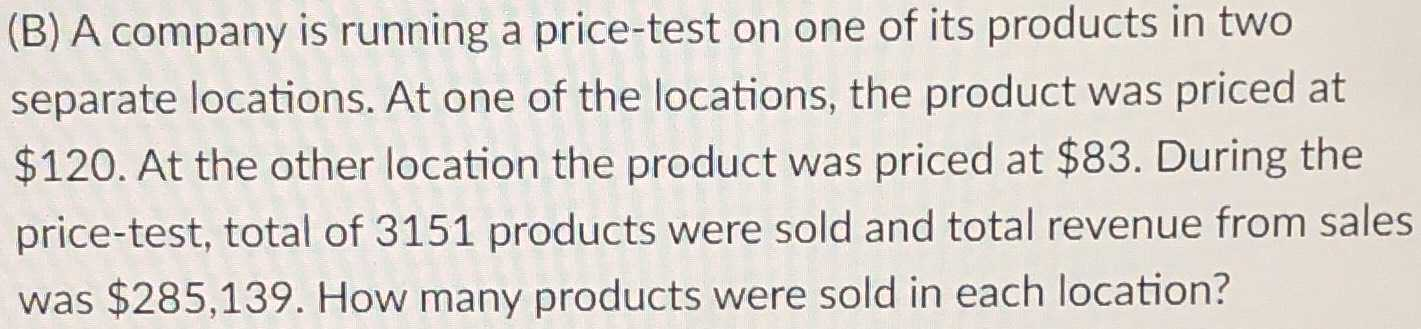### ¿Todavía tienes preguntas de matemáticas?

Pregunte a nuestros tutores expertos
Algebra
PreguntaA company is running a price-test on one of its products in two separate locations. At one of the locations, the product was priced at $$\ 120$$ . At the other location the product was priced at $$\ 83$$ . During the price-test, total of $$3151$$ products were sold and total revenue from sales was $$\ 285,139$$ . How many products were sold in each location?

$$\left[ \begin{array} { c } { x + y = 3151 } \\ { 120 x + 83 y = 285139 } \end{array} \right]$$

Isolate $$x$$ for $$x + y = 3151 : x = 3151 - y$$

Substitute $$x = 3151 - y$$

$$[ 120 ( 3151 - y ) + 83 y = 285139 ]$$

Simplify

$$[ 378120 - 37 y = 285139 ]$$

Isolate $$y$$ for $$378120 - 37 y = 285139 : y = 2513$$

For $$x = 3151 - y$$

Substitute $$y = 2513$$

$$x = 3151 - 2513$$

Simplify

$$x = 638$$

The solutions to the system of equations are:

$$x = 638 , y = 2513$$

Solución
View full explanation on CameraMath App.